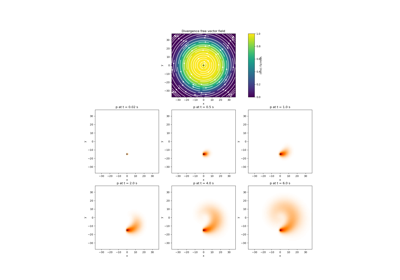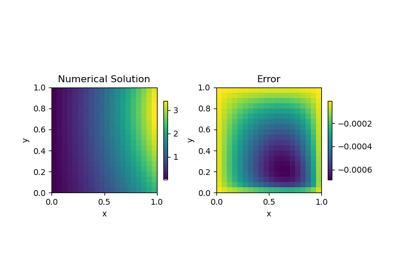# discretize.base.BaseRegularMesh#class discretize.base.BaseRegularMesh(shape_cells, origin=None, orientation=None, reference_system=None, **kwargs)[source]#

Base Regular mesh class for the discretize package.

The BaseRegularMesh class does all the basic counting and organizing you wouldn’t want to do manually. BaseRegularMesh is a class that should always be inherited by meshes with a regular structure; e.g. TensorMesh, CylindricalMesh, TreeMesh or CurvilinearMesh.

Parameters:
shape_cellsarray_like of int

number of cells in each dimension

originarray_like of float, optional

origin of the bottom south west corner of the mesh, defaults to 0.

orientationdiscretize.utils.Identity or array_like of float, optional

Orientation of the three major axes of the mesh; defaults to Identity. If provided, this must be an orthogonal matrix with the correct dimension.

reference_system{‘cartesian’, ‘cylindrical’, ‘spherical’}

Can also be a shorthand version of these, e.g. {‘car[t]’, ‘cy[l]’, ‘sph’}

Attributes

 axis_u Orientation of the first axis. axis_v Orientation of the second axis. axis_w Orientation of the third axis. dim The dimension of the mesh (1, 2, or 3). edge_tangents Unit tangent vectors for all mesh edges. face_normals Unit normal vectors for all mesh faces. n_cells Total number of cells in the mesh. n_edges Total number of edges in the mesh. n_edges_per_direction The number of edges in each direction. n_edges_x Number of x-edges in the mesh. n_edges_y Number of y-edges in the mesh. n_edges_z Number of z-edges in the mesh. n_faces Total number of faces in the mesh. n_faces_per_direction The number of faces in each axis direction. n_faces_x Number of x-faces in the mesh. n_faces_y Number of y-faces in the mesh. n_faces_z Number of z-faces in the mesh. n_nodes Total number of nodes in the mesh. orientation Rotation matrix defining mesh axes relative to Cartesian. origin Origin or 'anchor point' of the mesh. reference_is_rotated Indicate whether mesh uses standard coordinate axes. reference_system Coordinate reference system. rotation_matrix Alias for orientation. shape_cells Number of cells in each coordinate direction. x0 Alias for the origin.

## Galleries and Tutorials using discretize.base.BaseRegularMesh#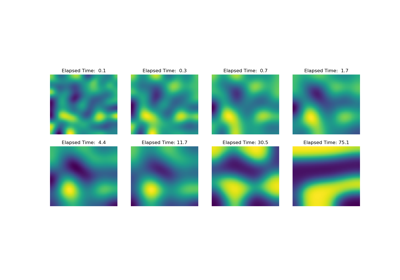Operators: Cahn Hilliard

Operators: Cahn Hilliard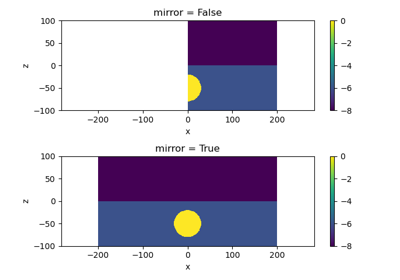Plot Mirrored Cylindrically Symmetric Model

Plot Mirrored Cylindrically Symmetric Model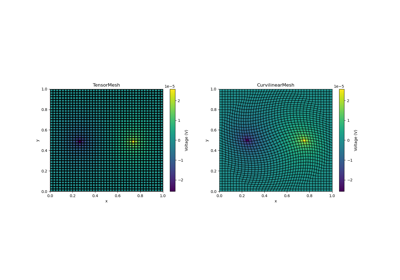Basic Forward 2D DC Resistivity

Basic Forward 2D DC ResistivityBasic: PlotImage

Basic: PlotImage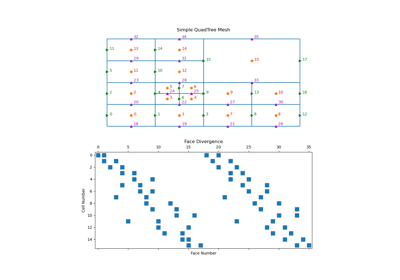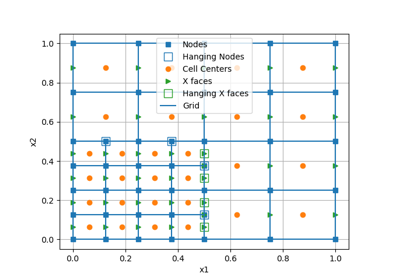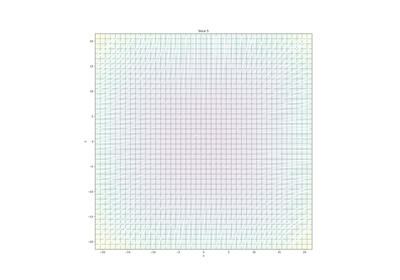Plotting: Streamline thickness

Plotting: Streamline thickness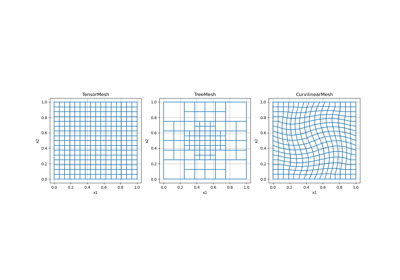Overview of Mesh Types

Overview of Mesh Types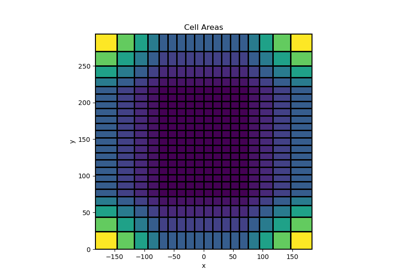Tensor meshes

Tensor meshes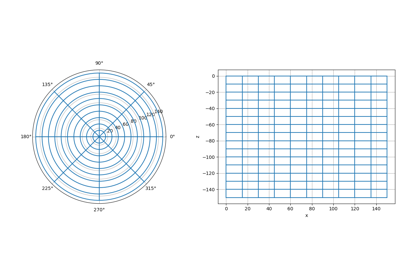Cylindrical meshes

Cylindrical meshes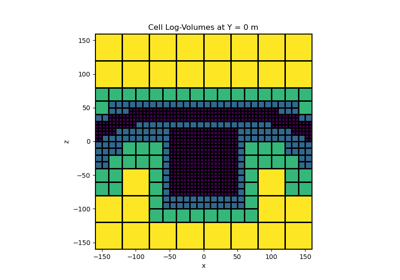Tree Meshes

Tree Meshes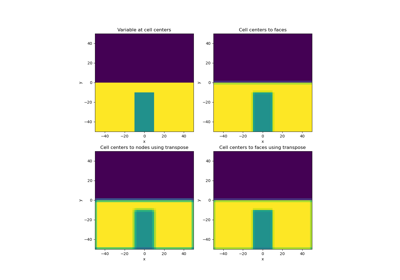Averaging Matricies

Averaging Matricies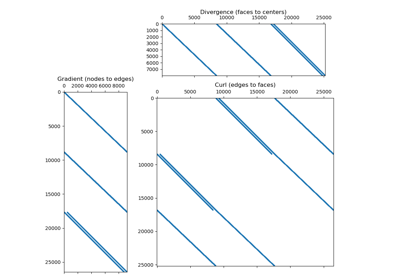Differential Operators

Differential Operators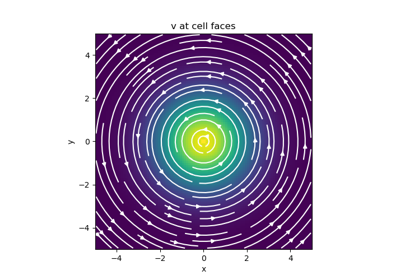Basic Inner Products

Basic Inner Products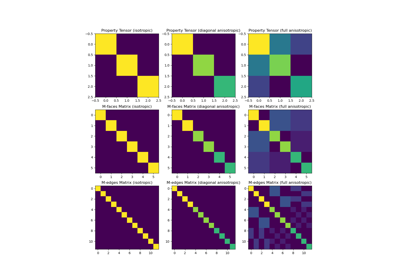Constitutive Relations

Constitutive Relations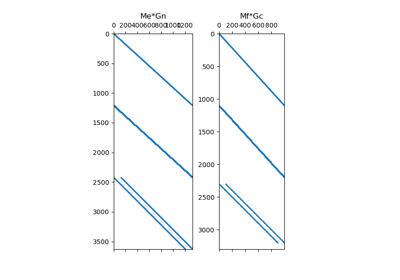Differential Operators

Differential Operators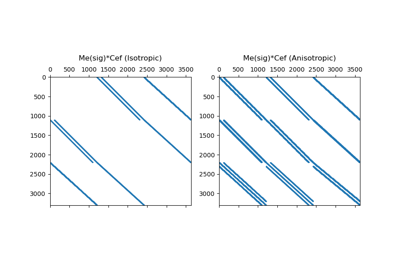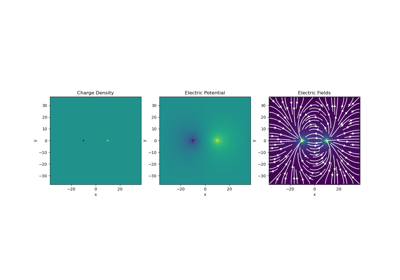Gauss’ Law of Electrostatics

Gauss' Law of Electrostatics